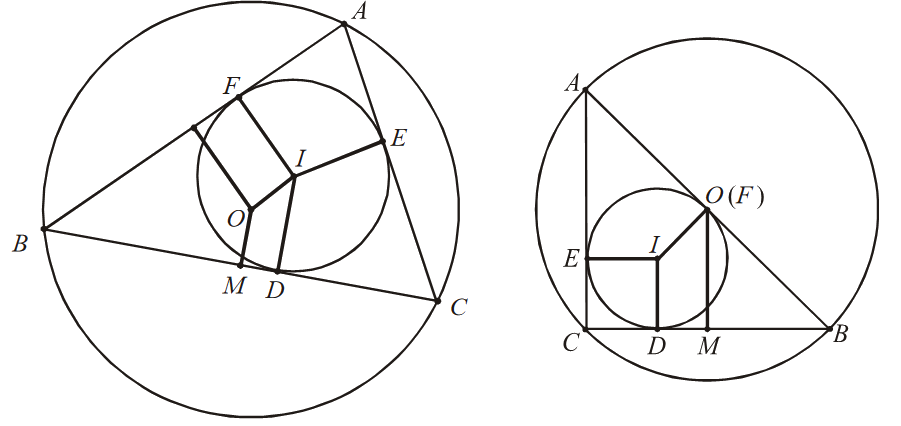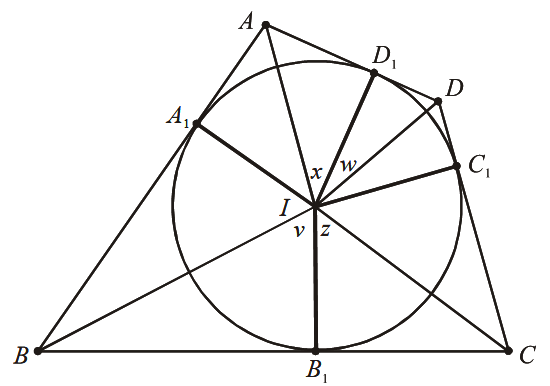# Trigonometry Set-8

Go back to  'SOLVED EXAMPLES'

Example - 19

Let $$ABC$$ be a nonobtuse triangle such that \begin{align}|AB|>|AC|\ \text{and}\ \angle B=45{}^\text{o}\end{align}. Let $$O$$ and $$I$$ denote the circumcenter and incenter of triangle $$ABC,$$ respectively. Suppose that \begin{align}\sqrt 2 |OI| = |AB| - |AC|\end{align}. Determine all the possible values of $$sin A$$.

Solution: Applying the extended law of sines to triangle $$ABC$$ yields \begin{align}a = 2R\sin A,\;b = 2R\sin B,\end{align} and \begin{align}c = 2R\sin C\end{align}. If incircle is tangent to side $$AB$$ at $$D.$$ Then \begin{align}|BD|\; = \frac{{c + a - b}}{2}\end{align}, and so \begin{align}r = \;|I\,D|\; = \;|B\,D|\tan \frac{B}{2}\end{align}. The half-angle formulas give

\begin{align}\tan \frac{B}{2} = \frac{{1 - \cos B}}{{\sin B}} = \frac{{1 - \frac{{\sqrt 2 }}{2}}}{{\frac{{\sqrt 2 }}{2}}} = \sqrt 2 - 1\end{align},and so

\begin{align}r = R\left( {\sqrt 2 - 1} \right)(\sin A + \sin C - \sin B)\end{align}

By Euler’s formula, \begin{align}|O\,I{|^2} = R(R - 2r)\end{align}, so we have

\begin{align}|O\,I{|^2} = {R^2} - 2Rr = {R^2}\left[ {1 - 2(\sin A + \sin C - \sin B)\left( {\sqrt 2 - 1} \right)} \right].\end{align}

Squaring both sides of the given equation \begin{align}\sqrt 2 |OI|\; = \;|AB| - |AC|\end{align} gives

\begin{align}|O\,I{|^2} = \frac{{{{(c - b)}^2}}}{2} = 2{R^2}{(\sin C - \sin B)^2}.\end{align}

Therefore

\begin{align}2{(\sin C - \sin B)^2} = 1 - 2(\sin A + \sin C - \sin B)\left( {\sqrt 2 - 1} \right)\end{align}

or

\begin{align}1 - 2{\left( {\sin C - \frac{{\sqrt 2 }}{2}} \right)^2} = 2\left( {\sin A + \sin C - \frac{{\sqrt 2 }}{2}} \right)\left( {\sqrt 2 - 1} \right) (*)\end{align}.

The addition and subtraction formulas give

\begin{align} \sin C & =\sin (180{}^\text{o}-B-A)=\sin (135{}^\text{o}-A) \\ & =\sin 135{}^\text{o}\cos A-\cos 135{}^\text{o}\sin A=\frac{\sqrt{2}(\sin A+\cos A)}{2} \\ \end{align}

and so

\begin{align}\sin C-\frac{\sqrt{2}}{2}=\frac{\sqrt{2}}{2}(\sin A+\cos A-1).\end{align}

Plugging the last equation into equation (*) yields

\begin{align}1-{{(\sin A+\cos A-1)}^{2}}=2\left( \sqrt{2}-1 \right)\left[ \sin A+\frac{\sqrt{2}}{2}(\sin A+\cos A-1) \right]\end{align},

Expanding both sides of the last equation gives

\begin{align} &1-{{(\sin A+\cos A)}^{2}}+2(\sin A+\cos A)-1 \\ & =\left( \sqrt{2}-1 \right)\left( 2+\sqrt{2} \right)\sin A+\left( 2-\sqrt{2} \right)(\cos A-1) \\ \end{align}

or

\begin{align}{{\sin }^{2}}A+{{\cos }^{2}}A+2\sin A\cos A=\left( 2-\sqrt{2} \right)\sin A+\sqrt{2}\cos A+\left( 2-\sqrt{2} \right).\end{align}

Consequently, we have

\begin{align}2\sin A\cos A-\left( 2-\sqrt{2} \right)\sin A-\sqrt{2}\cos A+\left( \sqrt{2}-1 \right)=0\ ;\end{align}

that is,

\begin{align}\left( \sqrt{2}\sin A-1 \right)\left( \sqrt{2}\cos A-\sqrt{2}+1 \right)=0.\end{align}

This implies that \begin{align}\sin A=\frac{\sqrt{2}}{2}\ \text{or}\ \cos A=1-\frac{\sqrt{2}}{2}\end{align}. Therefore, the answer to the problem is

\begin{align}\sin A=\frac{\sqrt{2}}{2}\ \text{or}\ \sin A=\sqrt{1-{{\cos }^{2}}A}=\frac{\sqrt{4\sqrt{2}-2}}{2}.\end{align}

Example - 20

A circle \begin{align}\omega \end{align} is inscribed in a quadrilateral $$ABCD.$$ Let I be the center of \begin{align}\omega \end{align}.

Suppose that

\begin{align}{{\left( |AI|+|DI| \right)}^{2}}+{{\left( |BI|+|CI| \right)}^{2}}{{\left( |AB|+|CD| \right)}^{2}}\end{align}

Prove that $$ABCD$$ is an isosceles trapezoid.

Solution: The key is to recognize that the given identity is a combination of equality cases of certain inequalities. By equal tangents, we have \begin{align}|AB| + |CD|\; = \;|AD| + |BC|\end{align} it and only if $$ABCD$$ has an incenter. We will prove that for a convex quadrilateral $$ABCD$$ with incenter $$I,$$ then

\begin{align}{(|AI| + |DI|)^2} + {(|BI| + |CI|)^2} \le {(|AB| + |CD|)^2} = {(|AD| + |BC|)^2}.(*)\end{align}

Equality holds if and only if \begin{align}AD||BC\;{\rm{and}}\;|AB|\; = \;|CD|\end{align}. Without loss of generality, we may assume that the inradius of $$ABCD$$ is $$I.$$As shown in figure. Let $${{A}_{1}},\ {{B}_{1}},\ {{C}_{1}}$$ and  $${{D}_{1}}$$ be the points of tangency.

Because circle $$\omega$$  is inscribed in $$ABCD,$$ we can set \begin{align}& \angle {{D}_{1}}IA=\angle AI{{A}_{1}}=x, \angle {{A}_{1}}I\,B=\angle BI{{B}_{1}}\,=y,{{B}_{1}}IC=LCI{{C}_{1}}=z,\angle {{C}_{1}}I\,D= \angle DI{{D}_{1}}=w,\ \text{and}\ x+y+z+w=180{}^\text{o},\\& \ \text{or}\ x+w=180{}^\text{o}-\,(y+z), \text{with} \;{0^0} < x,\;y,\;z,\;w < {90^0}.\end{align}

$$\text{Then} \;|AI|\ =\sec x,\ |BI|\ =\sec \,y,\ |CI|\ =\sec z,|DI|=\sec w,\ |AD|\ =\ |A{{D}_{1}}|+|{{D}_{1}}D|\ = \tan x+\tan w,\ \text{and}\ |BC|\ =|B{{B}_{1}}|+|{{B}_{1}}C|\ =\tan y+\tan z.\\\text{Inequality (*) becomes}$$

${{(\sec x+\sec w)}^{2}}+{{(\sec y+\sec z)}^{2}}\le {{(\tan x+\tan y+\tan z+\tan w)}^{2}}$

Expanding both sides of above inequality and applying the identity \begin{align}{\sec ^2}x = 1 + {\tan ^2}x\end{align} gives

\begin{align} 4 &+ 2(\sec x\sec w + \sec y\sec z)\\ & \le 2\tan x\tan y + 2\tan x\tan z + 2\tan x\tan w\\ & + 2\tan y\tan z + 2\tan y\tan w + 2\tan z\tan w \end{align}

or

\begin{align} & 2 + \sec x\sec w + \sec y\sec z\\ & \le \tan x\tan w + \tan y\tan z + (\tan x + \tan w)(\tan y + \tan z) \end{align}.

Note that by the addition and subtraction formulas.

\begin{align}1 - \tan x\tan w = \frac{{\cos x\cos w - \sin x\sin w}}{{\cos x\cos w}} = \frac{{\cos (x + w)}}{{\cos x\cos w}}\end{align}

Hence,

\begin{align}1 - \tan x\tan w + \sec y\sec z = \frac{{1 + \cos \,(y + z)}}{{\cos y\cos z}}\end{align}

Similarly,

\begin{align}1 - \tan y\tan z + \sec y\sec z = \frac{{1 + \cos (y + z)}}{{\cos y\cos z}}\end{align}

Adding the last two equations gives

\begin{align} & 2 + \sec x\sec w + \sec y\sec z - \tan x\tan w - \tan y\tan z\\ & = \frac{{1 + \cos (x + w)}}{{\cos x\cos w}} + \frac{{1 + \cos (y + z)}}{{\cos y\cos z}} \end{align}

It suffices to show that

\begin{align}\frac{{1 + \cos (x + \omega )}}{{\cos x\cos \omega }} + \frac{{1 + \cos (y + z)}}{{\cos y\cos z}} \le (\tan x + \tan \omega )(\tan y + \tan z)\end{align}

or

\begin{align}s + t \le \;(\tan x + \tan w)(\tan y + \tan z),\end{align}

after setting \begin{align}s = \frac{{1 + \cos (x + w)}}{{\cos x\cos w}}\;{\rm{and}}\;t = \frac{{1 + \cos (y + z)}}{{\cos x\cos w}}\end{align}. By the addition and subtraction formulas, we have

Similarly,

\begin{align}\tan y + \tan z = \frac{{\sin (y + z)}}{{\cos y\cos z}} = \frac{{\sin (x + w)}}{{\cos y\cos z}},\end{align}

because $$x + w = {180^0} - (y + z)$$. It follows that

\begin{align} & (\tan x + \tan w)(\tan y + \tan z)\\ & = \frac{{{{\sin }^2}(x + w)}}{{\cos x\cos y\cos z\cos w}} = \frac{{1 - {{\cos }^2}(x + w)}}{{\cos x\cos y\cos z\cos w}}\\ & = \frac{{[1 - \cos (x + w)][1 + \cos (x + w)]}}{{\cos x\cos y\cos z\cos w}}\\ & = \frac{{[1 + \cos (y + z)][1 + \cos (x + \omega )]}}{{\cos x\cos y\cos z\cos \omega }} = st. \end{align}

The desired inequality becomes \begin{align}s + t \le st\;{\rm{or}}\;(1 - s)(1 - t) = 1 - s - t + st \ge 1\end{align}. It suffices to show that \begin{align}1 - s \ge 1\;{\rm{and}}\;1 - t \ge 1.\end{align} By symmetry, we have only to show that \begin{align}1 - s \ge 1:\end{align} that is.

\begin{align}\frac{{1 + \cos (x + w)}}{{\cos x\cos w}} \ge 2.\end{align}

Multiplying both sides of the inequality by and applying the addition and subtraction formulas gives

\begin{align}1 + \cos x\cos w - \sin x\sin w \ge 2\cos x\cos w,\end{align}

or

\begin{align}1 \ge \cos x\cos w + \sin x\sin w = \cos (x - w),\end{align} which is evident. Equality holds if and only if \begin{align}x = w\end{align}. Therefore, inequality (*) is true, with equality if and only if \begin{align}x = \omega \;{\rm{and}}\;y = z\end{align}, which happens precisely when \begin{align}AD||BC\;{\rm{and}}\;|AB|\; = \;|CD|\end{align}, as was to be shown.

Learn from the best math teachers and top your exams

• Live one on one classroom and doubt clearing
• Practice worksheets in and after class for conceptual clarity
• Personalized curriculum to keep up with school